Mobile

# OpenGL Tutorial for Android: Building A Mandelbrot Set Generator

Asa is a computer science and math graduate with 6+ years of experience using Java, Python, and C++. He likes to work on machine learning.

OpenGL is a powerful cross-platform API that allows for very close access to the system’s hardware in a variety of programming environments.

So, why should you use it?

It provides a very low level processing for graphics in both 2D and 3D. In general, this will avoid any clunk that we have because of interpreted or high level programming languages. More importantly, though, it also provides hardware level access to a key feature: GPU.

GPU can significantly speed up many applications, but it has a very specific role in a computer. GPU cores are in fact slower than CPU cores. If we were to run a program that is notably serial with no concurrent activity, then it will almost always be slower on a GPU core than a CPU core. The main difference is that GPU supports massive parallel processing. We can create small programs called shaders that will run effectively on hundreds of cores at once. This means that we can take tasks that are otherwise incredibly repetitive and run them simultaneously.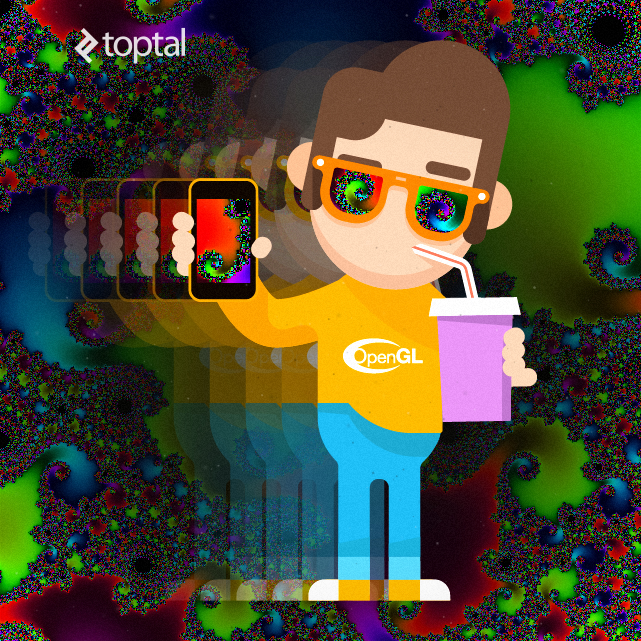In this article, we will be building a simple Android application that uses OpenGL to render its content on screen. Before we begin, It is important that you are already familiar with the knowledge of writing Android applications and syntax of some C-like programming language. The entire source code of this tutorial is available on GitHub.

## OpenGL Tutorial and Android

To demonstrate the power of OpenGL, we’ll be writing a relatively basic application for an Android device. Now, OpenGL on Android is distributed under a subset called OpenGL for Embedded Systems (OpenGL ES). We can essentially just think of this as a stripped down version of OpenGL, though the core functionality that is needed will still be available.

Instead of writing a basic “Hello World”, we’ll be writing a deceivingly simple application: a Mandelbrot set generator. The Mandelbrot set is based in the field of complex numbers. Complex analysis is a beautifully vast field, so we’ll be focusing on the visual result more than the actual math behind it.

With OpenGL, building a Mandelbrot set generator is easier than you think!

### Version Support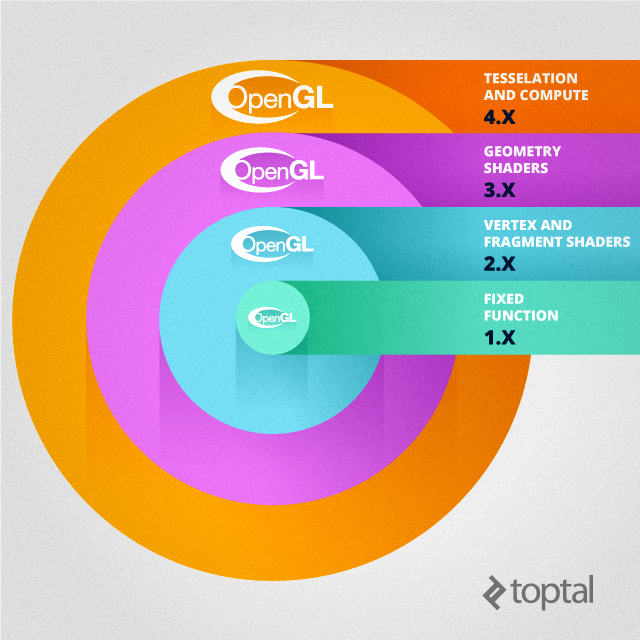When we are making the application, we want to make sure it is only distributed to those with proper OpenGL support. Start by declaring the use of OpenGL 2.0 in the manifest file, between the manifest declaration and application:

``````<uses-feature android:glEsVersion="0x00020000" android:required="true" />
``````

At this point, support for OpenGL 2.0 is ubiquitous. OpenGL 3.0 and 3.1 are gaining in compatibility, but writing for either will leave out roughly 65% of devices, so only make the decision if you are certain you’ll need additional functionality. They can be implemented by setting the version to ‘0x000300000’ and ‘0x000300001’ respectively.

## Application Architecture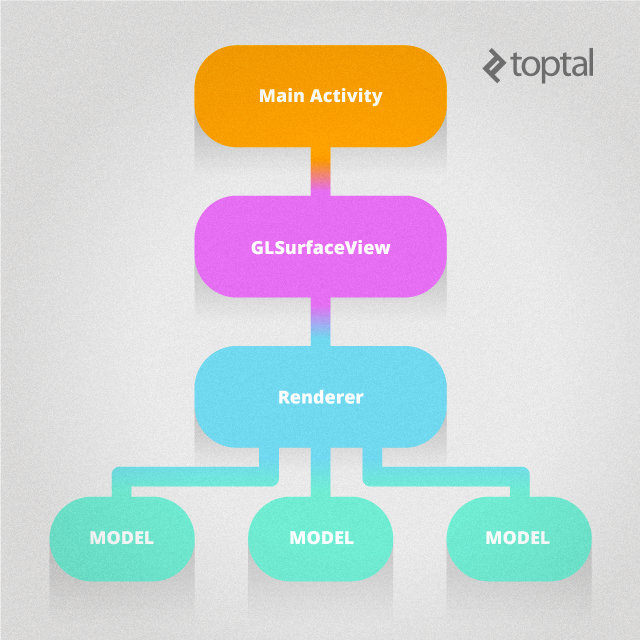When making this OpenGL application on Android, you’ll generally have three main classes that are used to draw the surface: your `MainActivity`, an extension of `GLSurfaceView`, and an implementation of a `GLSurfaceView.Renderer`. From there, we will create various Models that will encapsulate drawings.

`MainActivity`, called `FractalGenerator` in this example, is essentially just going to instantiate your `GLSurfaceView` and route any global changes down the line. Here’s an example that is essentially going to be your boilerplate code:

``````public class FractalGenerator extends Activity {

private GLSurfaceView mGLView;

@Override
public void onCreate(Bundle savedInstanceState) {
super.onCreate(savedInstanceState);

//Create and set GLSurfaceView
mGLView = new FractalSurfaceView(this);
setContentView(mGLView);
}

//[...]

@Override
protected void onPause() {
super.onPause();
mGLView.onPause();
}

@Override
protected void onResume() {
super.onResume();
mGLView.onResume();
}

}
``````

This is also going to be the class in which you will want to put any other activity level modifiers, (such as immersive fullscreen).

One class deeper, we have an extension of `GLSurfaceView`, which will act as our primary view. In this class, we set the version, set up a Renderer, and control for touch events. In our constructor, we need only to set the OpenGL version with `setEGLContextClientVersion(int version)` and also create and set our renderer:

``````public FractalSurfaceView(Context context){
super(context);

setEGLContextClientVersion(2);

mRenderer = new FractalRenderer();
setRenderer(mRenderer);
}
``````

Additionally, we can set attributes like the render mode with `setRenderMode(int renderMode)`. Because generating a Mandelbrot set can be very costly, we’ll be using `RENDERMODE_WHEN_DIRTY`, which will only render the scene at initialization and when explicit calls are made to `requestRender()`. More options for settings can be found in the `GLSurfaceView` API.

After we have the constructor, we’ll probably want to override at least one other method: `onTouchEvent(MotionEvent event)`, which can be used for general touch based user input. I’m not going to go into too much detail here, as that isn’t the main focus of the lesson.

Finally, we get down to our Renderer, which will be where most of the work for lighting or perhaps changes in scene happen. First, we’ll have to look a little bit into how matrices work and operate in the graphics world.

## Quick Lesson in Linear Algebra

OpenGL relies heavily on the use of matrices. Matrices are a wonderfully compact way of representing sequences of generalized changes in coordinates. Normally, they allow us to do arbitrary rotations, dilations/contractions, and reflections, but with a little bit of finesse we can also do translations. Essentially, this all means that you can easily perform any reasonable change you want, including moving a camera or making an object grow. By multiplying our matrices by a vector representing our coordinate, we can effectively produce the new coordinate system.

The Matrix class provided by OpenGL gives a number of ready-made ways of computing matrices we’ll need, but understanding how they work is a smart idea even when working with simple transformations.

First, we can go over why we will be using four dimensional vectors and matrices to deal with coordinates. This actually goes back to the idea of finessing our use of coordinates to be able to do translations: while a translation in 3D space is impossible using just three dimensions, adding a fourth dimension enables the ability.

To illustrate this, we can use a very basic general scale/translation matrix: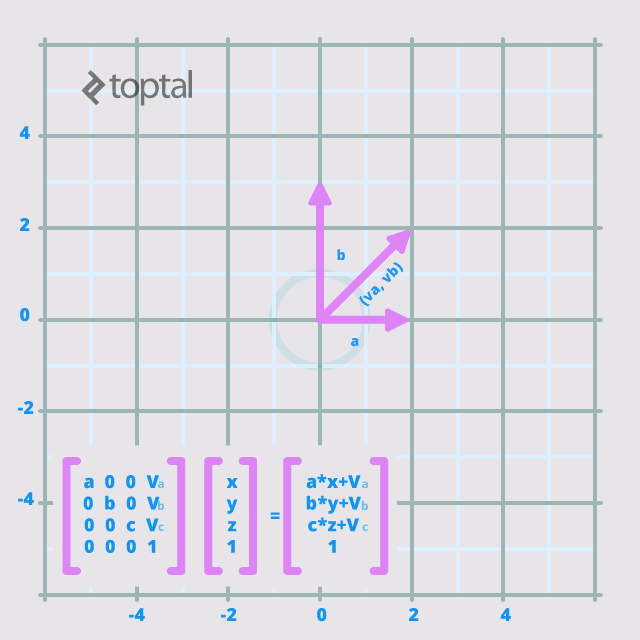As an important note, OpenGL matrices are column-wise, so this matrix would be written as `{a, 0, 0, 0, 0, b, 0, 0, 0, 0, c, 0, v_a, v_b, v_c, 1}`, which is perpendicular to how it usually will be read. This can be rationalized by ensuring that vectors, which appear in multiplication as a column, have the same format as matrices.

## Back to the Code

Armed with this knowledge of matrices, we can go back to designing our Renderer. Usually, we’ll create a matrix in this class that is formed from the product of three matrices: Model, View, and Projection. This would be called, appropriately, an MVPMatrix. You can learn more about the specifics here, as we are going to be using a more basic set of transformations—the Mandelbrot set is a 2 dimensional, fullscreen model, and it doesn’t really require the idea of a camera.

First, let’s set up the class. We’ll need to implement the required methods for the Renderer interface: `onSurfaceCreated(GL10 gl, EGLConfig config)`, `onSurfaceChanged(GL10 gl, int width, int height)`, and `onDrawFrame(GL10 gl)`. The full class will end up looking something like this:

``````public class FractalRenderer implements GLSurfaceView.Renderer {

//Provide a tag for logging errors
private static final String TAG = "FractalRenderer";

//Create all models
private Fractal mFractal;

//Transformation matrices
private final float[] mMVPMatrix = new float;

//Any other private variables needed for transformations

@Override
public void onSurfaceCreated(GL10 unused, EGLConfig config) {
// Set the background frame color
GLES20.glClearColor(0.0f, 0.0f, 0.0f, 1.0f);

//Instantiate all models
mFractal = new Fractal();
}

@Override
public void onDrawFrame(GL10 unused) {
//Clear the frame of any color information or depth information
GLES20.glClear(GLES20.GL_COLOR_BUFFER_BIT | GLES20.GL_DEPTH_BUFFER_BIT);

//Create a basic scale/translate matrix
float[] mMVPMatrix = new float[]{
-1.0f/mZoom,  0.0f,                0.0f,    0.0f,
0.0f,        1.0f/(mZoom*mRatio), 0.0f,    0.0f,
0.0f,        0.0f,                1.0f,    0.0f,
-mX,         -mY,                0.0f,    1.0f};

//Pass the draw command down the line to all models, giving access to the transformation matrix
mFractal.draw(mMVPMatrix);
}

@Override
public void onSurfaceChanged(GL10 unused, int width, int height) {
//Create the viewport as being fullscreen
GLES20.glViewport(0, 0, width, height);

//Change any projection matrices to reflect changes in screen orientation
}

//Other public access methods for transformations

}
``````

There are also two utility methods used in the provided code, `checkGLError` and `loadShaders` to assist in debugging and the use of shaders.

In all of this, we keep passing the chain of command down the line to encapsulate the different parts of the program. We’ve finally gotten down to the point where we can write what our program actually does, instead of how we can make theoretical changes to it. When doing this, we need to make a model class that contains the information that needs to be displayed for any given object in the scene. In complex 3D scenes, this could be an animal or a teakettle, but we’re going to be doing a fractal as a far simpler 2D example.

In Model classes, we write the entire class—there are no superclasses that must be used. We need only to have a constructor and some sort of draw method that takes any parameters in.

This said, there are still a number of variables we will need to have that are essentially boilerplate. Let’s take a look at the exact constructor used in the Fractal class:

``````public Fractal() {
// initialize vertex byte buffer for shape coordinates
ByteBuffer bb = ByteBuffer.allocateDirect(
// (# of coordinate values * 4 bytes per float)
squareCoords.length * 4);
bb.order(ByteOrder.nativeOrder());
vertexBuffer = bb.asFloatBuffer();
vertexBuffer.put(squareCoords);
vertexBuffer.position(0);

// initialize byte buffer for the draw list
ByteBuffer dlb = ByteBuffer.allocateDirect(
// (# of coordinate values * 2 bytes per short)
drawOrder.length * 2);
dlb.order(ByteOrder.nativeOrder());
drawListBuffer = dlb.asShortBuffer();
drawListBuffer.put(drawOrder);
drawListBuffer.position(0);

// create empty OpenGL Program
mProgram = GLES20.glCreateProgram();

// create OpenGL program executables
}
``````

Quite a mouthful, isn’t it? Luckily, this is a part of the program that you won’t have to change at all, save the name of the model. Provided you change the class variables appropriately, this should work well for basic shapes.

To discuss parts of this, let’s look at some variable declarations:

``````static float squareCoords[] = {
-1.0f,  1.0f, 0.0f,   // top left
-1.0f, -1.0f, 0.0f,   // bottom left
1.0f, -1.0f, 0.0f,   // bottom right
1.0f,  1.0f, 0.0f }; // top right

private final short drawOrder[] = { 0, 1, 2, 0, 2, 3 }; // order to draw vertices
``````

In `squareCoords`, we specify all coordinates of the square. Note that all coordinates on the screen are represented as a grid with `(-1,-1)` in the bottom left and `(1,1)` in the top right.

In `drawOrder`, we specify the order of the coordinates based on triangles that would make up the square. Particularly for consistency and speed, OpenGL uses triangles to represent all surfaces. To make a square, simply cut down a diagonal (in this case, `0` to `2`) to give two triangles.

In order to add both of these to the program, you first must convert them to a raw byte buffer to directly interface the contents of the array with the OpenGL interface. Java stores arrays as objects that contain additional information that is not directly compatible with the pointer based C arrays that OpenGL’s implementation uses. To remedy this, `ByteBuffers` are used, which store access to the raw memory of the array.

After we have put in the data for the vertices and draw order, we must create our shaders.

When creating a model, two shaders must be made: a Vertex Shader and a Fragment (Pixel) Shader. All shaders are written in GL Shading Language (GLSL), which is a C-based language with the addition of a number of built in functions, variable modifiers, primitives, and default input/output. On Android, these will be passed as final Strings through `loadShader(int type, String shaderCode)`, one of the two resource methods in the Renderer. Let’s first go over the different types of qualifiers:

• `const` : Any final variable can be declared as a constant so its value can be stored for easy access. Numbers like π can be declared as constants if they are used frequently throughout the shader. It is likely that the compiler will automatically declare unmodified values as constants, depending on the implementation.
• `uniform`: Uniform variables are ones that are declared constant for any single rendering. They are used essentially as static arguments to your shaders.
• `varying`: If a variable is declared as varying and is set in a vertex shader, then it is linearly interpolated in the fragment shader. This is useful for creating any sort of gradient in color and is implicit for depth changes.
• `attribute`: Attributes can be thought of as non-static arguments to a shader. They denote the set of inputs that are vertex specific and will only appear in Vertex Shaders.

In addition, we should discuss two other types of primitives that have been added:

• `vec2`, `vec3`, `vec4`: Floating point vectors of given dimension.
• `mat2`, `mat3`, `mat4`: Floating point matrices of given dimension.

Vectors can be accessed by their components `x`, `y`, `z`, and `w` or `r`, `g`, `b`, and `a`. They can also generate any size vector with multiple indices: for `vec3 a`, `a.xxyz` returns a `vec4` with the corresponding values of `a`.

Matrices and vectors can also be indexed as arrays, and matrices will return a vector with only one component. This means that for `mat2 matrix`, `matrix.a` is valid and will return `matrix`. When working with these, remember that they act as primitives, not objects. For instance, consider the following code:

``````vec2 a = vec2(1.0,1.0);
vec2 b = a;
b.x=2.0;
``````

This leaves `a=vec2(1.0,1.0)` and `b=vec2(2.0,1.0)`, which is not what one would expect from object behavior, where the second line would give `b` a pointer to `a`.

In the Mandelbrot Set, the majority of the code will be in the fragment shader, which is the shader that runs on every pixel. Nominally, vertex shaders work on every vertex, including attributes that will be on a per vertex basis, like changes to color or depth. Let’s take a look at the extremely simple vertex shader for a fractal:

``````private final String vertexShaderCode =
"attribute vec4 vPosition;" +
"void main() {" +
"  gl_Position = vPosition;" +
"}";
``````

In this, `gl_Position` is an output variable defined by OpenGL to record the coordinates of a vertex. In this case, we pass in a position for each vertex to which we set `gl_Position`. In most applications, we would multiply `vPosition` by an `MVPMatrix`, transforming our vertices, but we want the fractal to always be full screen. All transformations will be done with a local coordinate system.

The Fragment Shader is going to be where most of the work is done to generate the set. We’ll set `fragmentShaderCode` to the following:

``````precision highp float;
uniform mat4 uMVPMatrix;

void main() {
//Scale point by input transformation matrix
vec2 p = (uMVPMatrix * vec4(gl_PointCoord,0,1)).xy;
vec2 c = p;

//Set default color to HSV value for black
vec3 color=vec3(0.0,0.0,0.0);

//Max number of iterations will arbitrarily be defined as 100. Finer detail with more computation will be found for larger values.
for(int i=0;i<100;i++){
//Perform complex number arithmetic
p= vec2(p.x*p.x-p.y*p.y,2.0*p.x*p.y)+c;

if (dot(p,p)>4.0){
//The point, c, is not part of the set, so smoothly color it. colorRegulator increases linearly by 1 for every extra step it takes to break free.
float colorRegulator = float(i-1)-log(((log(dot(p,p)))/log(2.0)))/log(2.0);
//This is a coloring algorithm I found to be appealing. Written in HSV, many functions will work.
color = vec3(0.95 + .012*colorRegulator , 1.0, .2+.4*(1.0+sin(.3*colorRegulator)));
break;
}
}
//Change color from HSV to RGB. Algorithm from https://gist.github.com/patriciogonzalezvivo/114c1653de9e3da6e1e3
vec4 K = vec4(1.0, 2.0 / 3.0, 1.0 / 3.0, 3.0);
vec3 m = abs(fract(color.xxx + K.xyz) * 6.0 - K.www);
gl_FragColor.rgb = color.z * mix(K.xxx, clamp(m - K.xxx, 0.0, 1.0), color.y);

gl_FragColor.a=1.0;
}
``````

Much of the code is merely the math and algorithm for how the set works. Note the use of several built in functions: `fract`, `abs`, `mix`, `sin`, and `clamp`, which all operate on vectors or scalars and return vectors or scalars. Additionally, `dot` is used which takes vector arguments and returns a scalar.

Now that we have our shaders set up for use, we have one last step, which is to implement the `draw` function in our model:

``````public void draw(float[] mvpMatrix) {
// Add program to OpenGL environment
GLES20.glUseProgram(mProgram);

// get handle to vertex shader's vPosition member
mPositionHandle = GLES20.glGetAttribLocation(mProgram, "vPosition");
mMVPMatrixHandle = GLES20.glGetUniformLocation(mProgram, "uMVPMatrix");

//Pass uniform transformation matrix to shader
GLES20.glUniformMatrix4fv(mMVPMatrixHandle, 1, false, mvpMatrix, 0);

GLES20.glEnableVertexAttribArray(mPositionHandle);
GLES20.glVertexAttribPointer(
mPositionHandle, COORDS_PER_VERTEX,
GLES20.GL_FLOAT, false,
vertexStride, vertexBuffer);

// Draw the square
GLES20.glDrawElements(
GLES20.GL_TRIANGLES, drawOrder.length,
GLES20.GL_UNSIGNED_SHORT, drawListBuffer);

// Disable vertex array
GLES20.glDisableVertexAttribArray(mPositionHandle);
FractalRenderer.checkGlError("Test");
}
``````

The function passes all arguments to the shaders, including the `uniform` transformation matrix and the `attribute` position.

After assembling all parts of the program, we can finally give it a run. Provided that proper touch support is handled, absolutely mesmerizing scenes will be painted: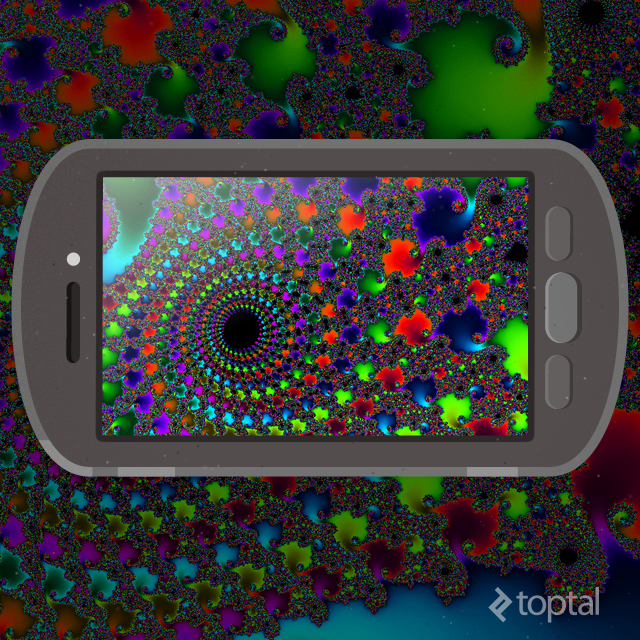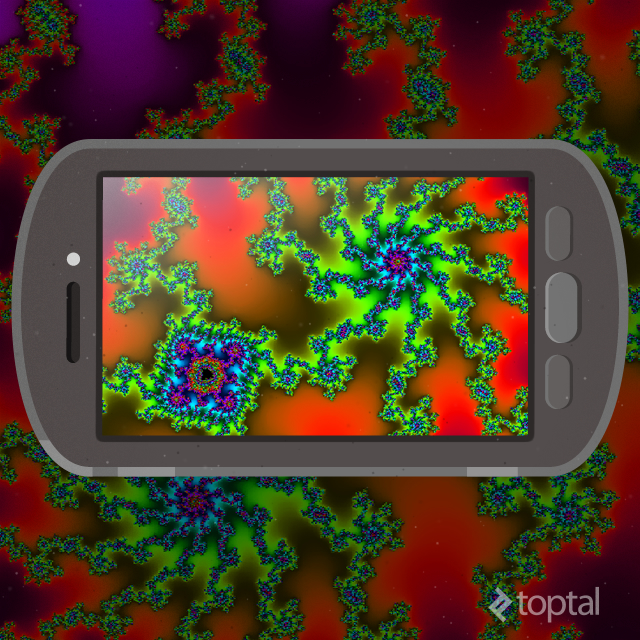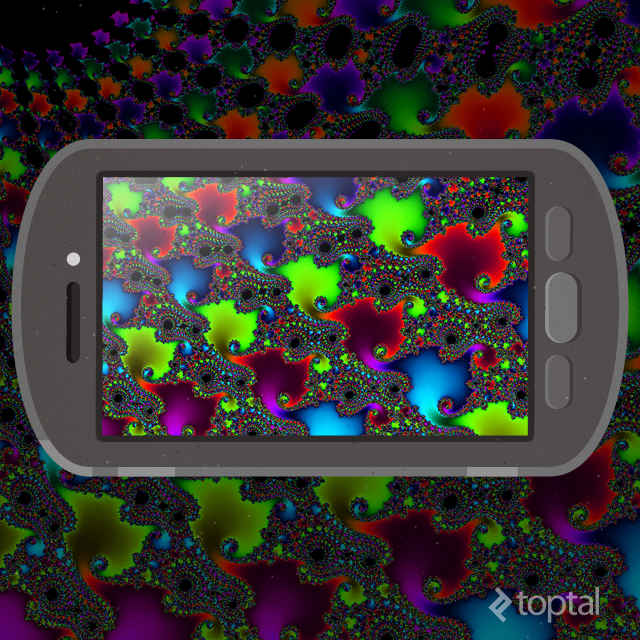## Floating Point Accuracy

If we zoom in a bit more, we start to notice a break down in the image: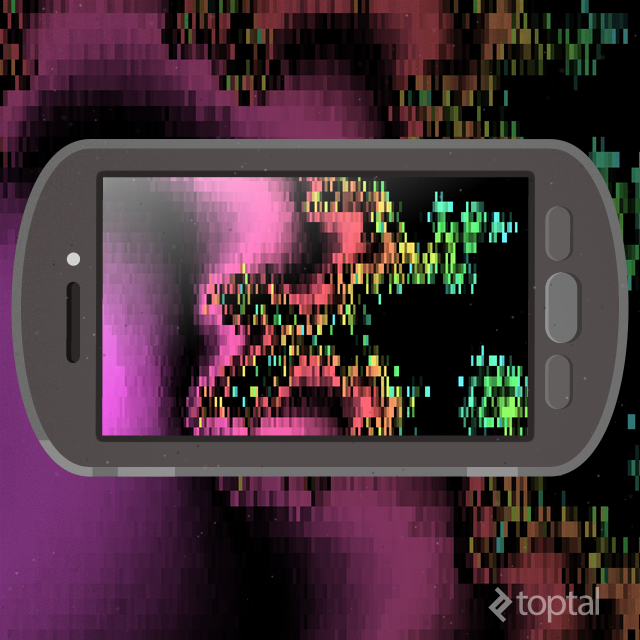This has absolutely nothing to do with the math of the set behind it and everything to do with the way numbers are stored and processed in OpenGL. While more recent support for `double` precision has been made, OpenGL 2.0 does not natively support anything more than `float`s. We specifically designated them to be the highest precision floats available with `precision highp float` in our shader, but even that isn’t good enough.

In order to get around this issue, the only way would be to emulate `double`s using two `float`s. This method actually comes within an order of magnitude of the actual precision of a natively implemented one, though there is a rather severe cost to speed. This will be left as an exercise to the reader, if one wishes to have a higher level of accuracy.

## Conclusion

With a few support classes, OpenGL can quickly sustain real time rendering of complex scenes. Creating a layout composed of a `GLSurfaceView`, setting its `Renderer`, and creating a model with shaders all culminated in the visualization of a beautiful mathematical structure. I hope that you’ll find as much interest in developing an OpenGL ES application!